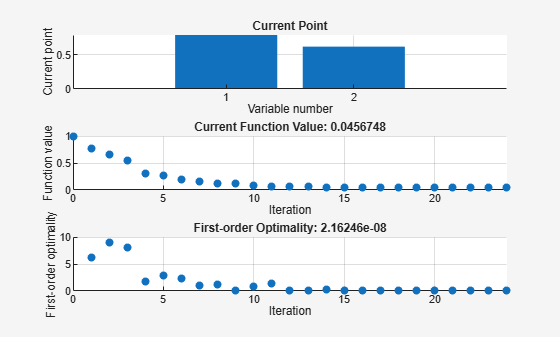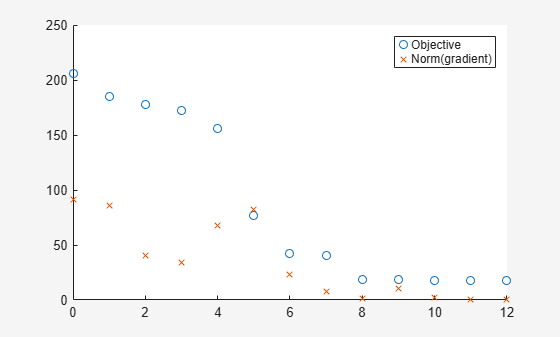## Plot Functions

### Plot Optimization During Execution

You can plot various measures of progress during the execution of a solver. Use the `PlotFcn` name-value argument of `optimoptions` to specify one or more plotting functions for the solver to call at each iteration. Pass a function handle, function name, or cell array of function handles or function names as the `PlotFcn` value.

Each solver has a variety of predefined plot functions available. For more information, see the `PlotFcn` option description on the function reference page for a solver.

You can also use a custom plot function, as shown in Create Custom Plot Function. Write a function file using the same structure as an output function. For more information on this structure, see Output Function and Plot Function Syntax.

### Use Predefined Plot Functions

This example shows how to use plot functions to view the progress of the `fmincon` "`interior-point"` algorithm. The problem is taken from Constrained Nonlinear Problem Using Optimize Live Editor Task or Solver.

Write the nonlinear objective and constraint functions, including their gradients. The objective function is Rosenbrock's function.

`type rosenbrockwithgrad`
```function [f,g] = rosenbrockwithgrad(x) % Calculate objective f f = 100*(x(2) - x(1)^2)^2 + (1-x(1))^2; if nargout > 1 % gradient required g = [-400*(x(2)-x(1)^2)*x(1)-2*(1-x(1)); 200*(x(2)-x(1)^2)]; end ```

Save this file as `rosenbrockwithgrad.m`.

The constraint function is that the solution satisfies `norm(x)^2 <= 1`.

`type unitdisk2`
```function [c,ceq,gc,gceq] = unitdisk2(x) c = x(1)^2 + x(2)^2 - 1; ceq = [ ]; if nargout > 2 gc = [2*x(1);2*x(2)]; gceq = []; end ```

Save this file as `unitdisk2.m`.

Create options for the solver that include calling three plot functions.

```options = optimoptions(@fmincon,Algorithm="interior-point",... SpecifyObjectiveGradient=true,SpecifyConstraintGradient=true,... PlotFcn={@optimplotx,@optimplotfval,@optimplotfirstorderopt});```

Create the initial point `x0 = [0,0]`, and set the remaining inputs to empty (`[]`).

```x0 = [0,0]; A = []; b = []; Aeq = []; beq = []; lb = []; ub = [];```

Call `fmincon`, including the options.

```fun = @rosenbrockwithgrad; nonlcon = @unitdisk2; x = fmincon(fun,x0,A,b,Aeq,beq,lb,ub,nonlcon,options)``````Local minimum found that satisfies the constraints. Optimization completed because the objective function is non-decreasing in feasible directions, to within the value of the optimality tolerance, and constraints are satisfied to within the value of the constraint tolerance. ```
```x = 1×2 0.7864 0.6177 ```

### Create Custom Plot Function

To create a custom plot function for an Optimization Toolbox™ solver, write a function using the syntax

```function stop = plotfun(x,optimValues,state) stop = false; switch state case "init" % Set up plot case "iter" % Plot points case "done" % Clean up plots % Some solvers also use case "interrupt" end end ```

Global Optimization Toolbox solvers use different syntaxes.

The software passes the `x`, `optimValues`, and `state` data to your plot function. For complete syntax details and a list of the data in the `optimValues` argument, see Output Function and Plot Function Syntax. You pass the plot function name to the solver using the `PlotFcn` name-value argument.

For example, the `plotfandgrad` helper function listed at the end of this example plots the objective function value and norm of the gradient for a scalar-valued objective function. Use the `ras` helper function listed at the end of this example as the objective function. The `ras` function includes a gradient calculation, so for efficiency, set the `SpecifyObjectiveGradient` name-value argument to `true`.

```fun = @ras; rng(1) % For reproducibility x0 = 10*randn(2,1); % Random initial point opts = optimoptions(@fminunc,SpecifyObjectiveGradient=true,PlotFcn=@plotfandgrad); [x,fval] = fminunc(fun,x0,opts)``````Local minimum found. Optimization completed because the size of the gradient is less than the value of the optimality tolerance. ```
```x = 2×1 1.9949 1.9949 ```
```fval = 17.9798 ```

The plot shows that the norm of the gradient converges to zero, as expected for an unconstrained optimization.

Helper Functions

This code creates the `plotfandgrad` helper function.

```function stop = plotfandgrad(x,optimValues,state) persistent iters fvals grads % Retain these values throughout the optimization stop = false; switch state case "init" iters = []; fvals = []; grads = []; case "iter" iters = [iters optimValues.iteration]; fvals = [fvals optimValues.fval]; grads = [grads norm(optimValues.gradient)]; plot(iters,fvals,"o",iters,grads,"x"); legend("Objective","Norm(gradient)") case "done" end end```

This code creates the `ras` helper function.

```function [f,g] = ras(x) f = 20 + x(1)^2 + x(2)^2 - 10*cos(2*pi*x(1) + 2*pi*x(2)); if nargout > 1 g(2) = 2*x(2) + 20*pi*sin(2*pi*x(1) + 2*pi*x(2)); g(1) = 2*x(1) + 20*pi*sin(2*pi*x(1) + 2*pi*x(2)); end end```